# Settling Chamber Design Notes | Study Environmental Engineering - Civil Engineering (CE)

## Civil Engineering (CE): Settling Chamber Design Notes | Study Environmental Engineering - Civil Engineering (CE)

The document Settling Chamber Design Notes | Study Environmental Engineering - Civil Engineering (CE) is a part of the Civil Engineering (CE) Course Environmental Engineering.
All you need of Civil Engineering (CE) at this link: Civil Engineering (CE)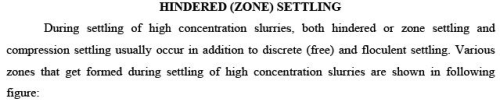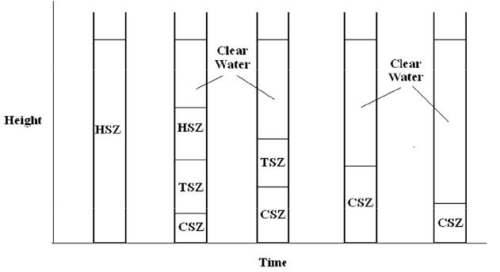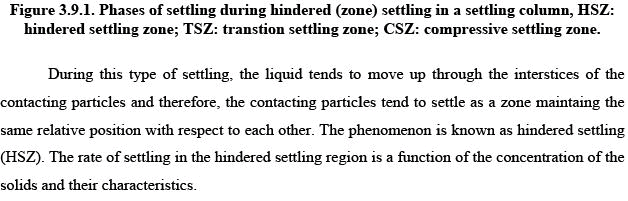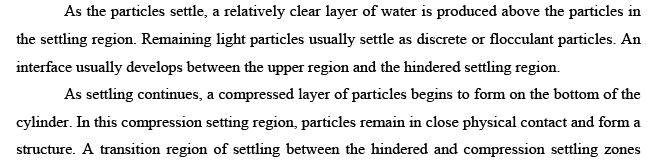gets formed. As the time progresses, first hindered and then transtion settling zones get removed and finally only clear water zone and compressed settling layer are only obtained . These methods are, however, seldom used in the design of treatment plants because of less concentration of slurries.

Area requirement based on single-batch test results.

For purposes of design, the final overflow rate selected should be based on a consideration of the following factors: 1) The area needed for clarification
2) The area needed for thickening, and
3) The rate of sludge withdrawal.

Column settling tests can be used to determine the area needed for the settling region directly. However, because the area required for thickening is usually greater than the area required for the settling, the rate of free settling rarely is the controlling factor. In the case of the activated-sludge process, where light fluffy floc particles are present, the free flocculant settling velocity of these particles could control the design.

AREA REQUIRED FOR THICKENING

Assume that a column of height Ho is filed with a suspension of solids of uniform concentration (Co). The rate at which the interface subsided is then equal to the slope of the curve at that point in time. According to the procedure, the area required for thickening is given by: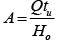(3.9.1)
Where, A is area required for sludge thickening (m)2, Q is the flow rate into tank (m3/s), Ho is the initial height of interface in column (m), tu is the time to each desired underflow concentration (s). The critical concentration controlling the solids handling capability of the tank occurs at a height H2 where the concentration is C2 . This point is determined by extending the tangents to the hindered settling and compression regions of the subsidence curve to the point of intersection and bisecting the angle thus formed.  The time t u can be determined as follows:

a) Construct a horizontal line at the depth Hu that corresponding to the depth at which the solids are at the desired underflow concentration Cu . The value of His determined using the following expression :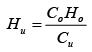(3.9.2)

(b) Construct a tangent to the settling curve at the point indicated by C2 . (c) Construct a vertical line from the point of intersection of the two lines drawn in steps 1 and 2 to the time axis to determine the value of tu . With this value tu , the area required for the thickening is computed using equation earlier. The area required for clarification is then determined. The larger of the two areas is the controlling value.

Problem 3.9.1: The settling curve shown in the following diagram was obtained for an activated sludge with an initial solid concentration Co of 3000 mg/l. The initial height of the interface in the settling column is 1 m. Determine the area required to yield a thickened solids concentration (Cu) of 15000 mg/l with a total flow of 3 m3/min. Also determine the solids loading (kg/m2.d) and the overflow rate (m3/m2.d).

Solution: [A] Determination of the area required for thickening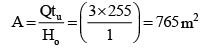A horizontal line is constructed at Hu=0.2 m which meets the settling curve at point C1. Tangents are drawn to the curve at point C1 and as well as at time t=0. Line bisecting the angle formed between the tangents meet the settling curve at point C2.  A tangent is further drawn to the settling curve at C2 (the mid-point of the region between hindered and compression settling). The intersection of the tangent at C2 and the line Hu=0.2 m determines tu=255 min.

Thus for tu=255 min, and the required area is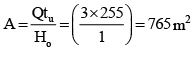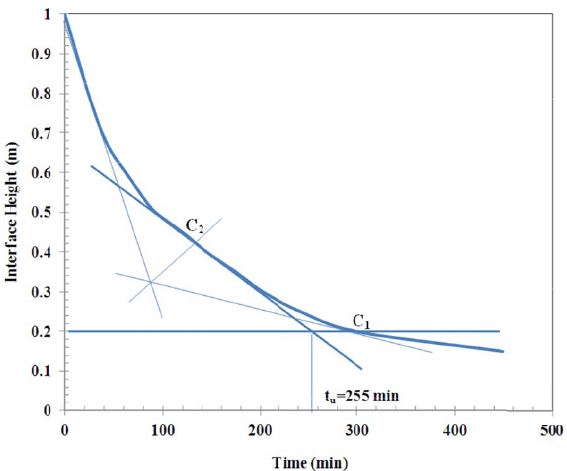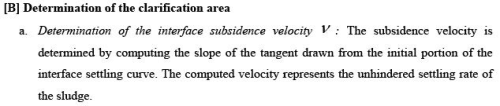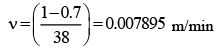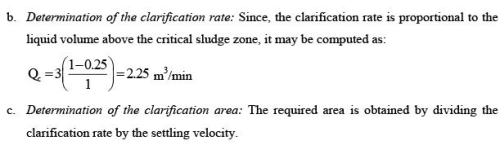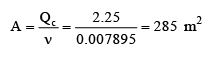The controlling area is the thickening area (765 m2) because it exceeds the area required for clarification (285 m2).

Solids, kg/d =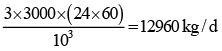Solids loading =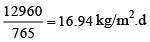Hydraulic loading rate =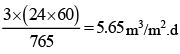The document Settling Chamber Design Notes | Study Environmental Engineering - Civil Engineering (CE) is a part of the Civil Engineering (CE) Course Environmental Engineering.
All you need of Civil Engineering (CE) at this link: Civil Engineering (CE)Use Code STAYHOME200 and get INR 200 additional OFF

## Environmental Engineering

14 videos|74 docs|70 tests

Track your progress, build streaks, highlight & save important lessons and more!

,

,

,

,

,

,

,

,

,

,

,

,

,

,

,

,

,

,

,

,

,

;